### MEMO 2018 pojedinačno problem 3

Kvaliteta:
Avg: 0,0
Težina:
Avg: 6,0

Let$ABC$ be an acute-angled triangle with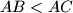$AB < AC$ and let$D$ be the foot of its altitude from$A$. Let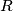$R$ and$Q$ be the centroids of triangles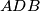$ADB$ and$ADC$ respectively. Let$P$ be a point on the line segment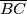$\overline{BC}$ such that the points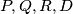$P, Q, R, D$ are concyclic.

Prove that the line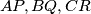$AP, BQ, CR$ are concurrent.

Izvor: Srednjoeuropska matematička olimpijada 2016, pojedinačno natjecanje, problem 3